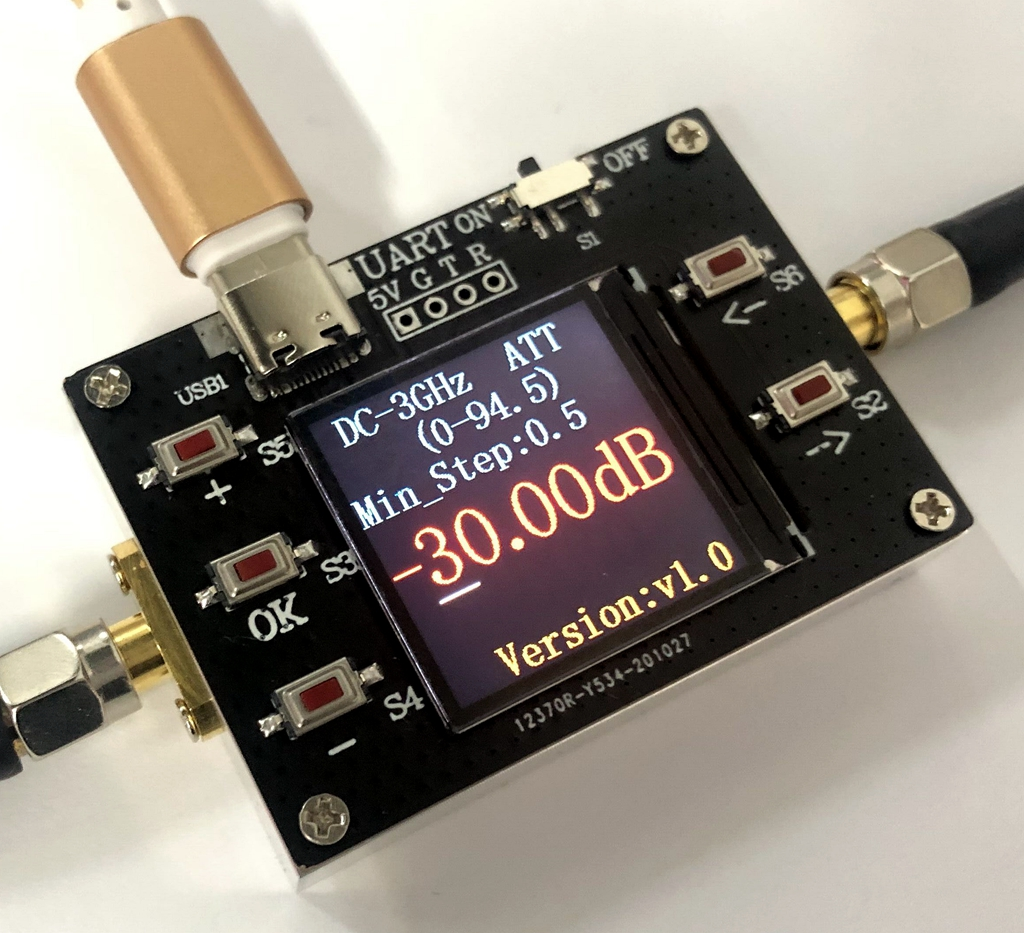## Friday, June 2, 2017

### Programming Multiple Channels in the Arduino - Radio-SkyPipe Project.

If you need a strip chart recorder for your Arduino project you might want to consider the Radio-SkyPipe.  The free version of Radio-SkyPipe will work for charting a single channel of data arriving through UDS (User Data Source) feature of RSP.  Typically you might plot one of the analog to digital converter (ADC) inputs on the Arduino. I introduced that here.  You might also be interested in how to connect to your Arduino with RSP over Ethernet or Wireless.

If you have the Pro version of RSP you can plot up to 8 channels and this post contains some sample code for the Arduino that can accomplish that. This code will work with the Uno or the Mega but of course, you have fewer analog inputs to work with on the Uno and this example is limited to 4 channels.

// Skeleton sketch for UDS connection to Radio-SkyPipe
// there are a lot of commented out print statements that
// can be helpful in debugging.

int POLL; // if =1 then data is polled by RSP using a GETD command
int STAT; // -1 = we were just stopped by a KILL command 0 = startup state 1 = INIT rcvd 2 = Ready to Go 3= Running

void setup() {
STAT == 0;
POLL == 0;
Serial.begin(9600); // connect to the serial port
//delay(1000);
//Serial.print("^^1002Arduino UDS");
//Serial.write(255);
}

int incomingByte;

void loop() {
// if we are pushing the data to RSP then we need to
// establish our timing for sending new data.
// here we are just doing a delay of 100ms to get a
// sample rate of about 10 samples / sec.
if (POLL == 0 && STAT ==3){
delay(100);
}

while (Serial.available() > 0) {

// read the oldest byte in the serial buffer:

// if it's an K we stop (KILL):
if (incomingByte == 'K') {
//Serial.write(255);
//Serial.println("Arduino UDS");
//GET PAST THE REST OF THE WORD by Reading it.
delay(10); // not sure why these delays were needed
delay(10);
delay(10);
incomingByte = 0;
STAT=-1 ;
}
// if it's a capital I run the INIT code if any
if (incomingByte == 'I' && STAT ==0) {
//INIT
// GET RID OF 'NIT'
delay(10);
delay(10);
delay(10);
incomingByte = 0;
STAT = 1 ;
//Serial.print("^^1002 INITIALIZED ");
//Serial.write(255);
}
// if it's an L (ASCII 76) RSP will POLL for data
if (incomingByte == 'L') {
POLL = 1;
// GET RID OF 'L'
delay(10);
incomingByte = 0;
//Serial.print("^^1002 POLLING ");
//Serial.write(255);
}

// H sets it to push
if (incomingByte == 'H') {
POLL = 0;
//Serial.print("^^1002 PUSHING ");
//Serial.write(255);
}

// if it's a C then Radio-SkyPipe is requesting number of channels
if (incomingByte == 'C') {
// change the last digit to = digit of channels of data (ex. 1)
delay(10);
Serial.print("^^20134");
Serial.write(255); // print result;
STAT = 2; // ready to go
}
if (incomingByte == 'A' ) {
// A means STAT was requested so send UDS ready message
delay(10);
Serial.print("^^1001");
Serial.write(255); // print result;;
// GET RID OF 'T'
delay(10);
incomingByte = 0;
STAT=3;

}
// if it's an D we should send data to RSP:
if (incomingByte == 'D' && POLL == 1 ) {
GETD() ;
}

if (STAT== -1){
STAT = 0;
}

}
// we are finished processing any incoming commands from the PC
// and we are not being polled so get a sample and send it
if (POLL == 0 && STAT == 3) {
GETD ();
}
}

// This is where data is fetched and sent on to RSP.

long int dat; //may have to change type to suit your data
void GETD(){
dat = analogRead(A0); // Replace this with a call to whatever data collection routine
Serial.print("#0"); // # followed by channel number of data
Serial.print(dat);
Serial.write(255);
dat = analogRead(A1); // Replace this with a call to whatever data collection routine
Serial.print("#0"); // # followed by channel number of data
Serial.print(dat);
Serial.write(255);
dat = analogRead(A2); // Replace this with a call to whatever data collection routine
Serial.print("#0"); // # followed by channel number of data
Serial.print(dat);
Serial.write(255);
dat = analogRead(A3); // Replace this with a call to whatever data collection routine
Serial.print("#0"); // # followed by channel number of data
Serial.print(dat);
Serial.write(255);
Serial.print("^^3001"); // This tells RSP to time stamp it
Serial.write(255); // all commands end with this character.
return;
}